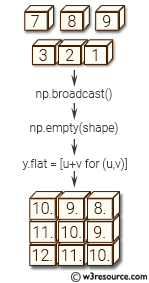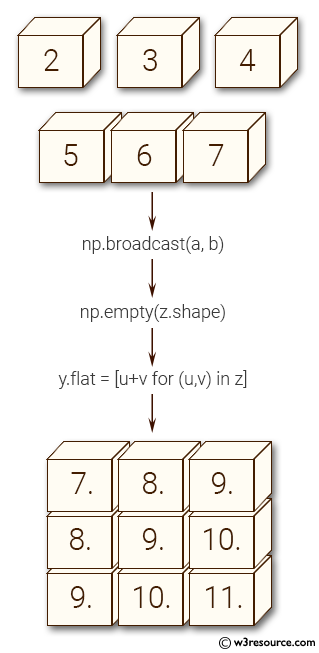# NumPy Array manipulation: broadcast() function

Syntax:

`class numpy.broadcast`Version: 1.15.0

Parameter:

Name Description Required /
Optional
in1, in2, . . . Input parameters. Required

Return value:

b [broadcast object] Broadcast the input parameters against one another, and return an object that encapsulates the result. Amongst others, it has shape and nd properties, and may be used as an iterator.

``````>>> import numpy as np
>>> a = np.array([, , ])
>>> b = np.array([5, 6, 7])
>>> y = np.empty(z.shape)
>>> y.flat = [u+v for (u,v) in z]
>>> y
array([[  7.,   8.,   9.],
[  8.,   9.,  10.],
[  9.,  10.,  11.]])
``````

``````>>> import numpy as np
>>> a = np.array([, , ])
>>> b = np.array([5, 6, 7])
>>> a+b
array([[ 7,  8,  9],
[ 8,  9, 10],
[ 9, 10, 11]])
``````

Pictorial Presentation:Python - NumPy Code Editor:

Previous: atleast_3d()